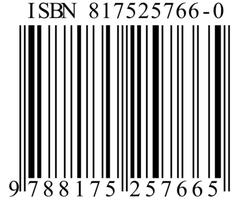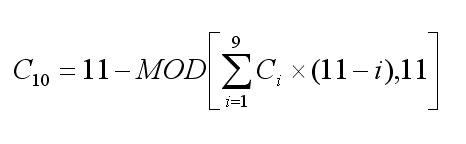# 国际标准书号（ISBN）10位ISBN校验码计算方法

1x7+2x5+3x6+4x0+5x0+6x3+7x8+8x7+9x9
= 7+10+18+0+0+18+56+56+81
= 246
= 242+4
= 22x11+4

13位ISBN校验码计算方法

13位ISBN的校验码计算规则是这样的：前12位数依次乘以1和3，然后求它们的和除以10的余数，最后用10减去这个余数，就得到了校验码。如果余数为0，则校验码为0.

9x1+7x3+8x1+7x3+5x1+6x3+0x1+0x3+3x1+8x3+7x1+9x3
= 9+21+8+21+5+18+0+0+3+24+7+27
= 143
= 140+3
= 14x10+3

10-3=7ISBN在国际上是一个非强制规定，就是说出版商完全可以出版没有ISBN的出版物。但是在中国，这却是一个强制性规定。没有书号，就不是合法出版物。这完全就是另外一个故事了。

[参考链接]

（完）

UPDATE （2007.6.1）

10位ISBN的另一种计算方法C10表示第10位校验码，C1-C9表示0~9位数字。

（完）

## 留言（18条）

`引用Frances的发言：`

00725就是右边条码的内容，可以直接从条码上读出书的价格，或者也可以先读出isbn，然后从数据库中调出价格。

`引用SO ME PIG的发言：`

9787300060347的后10位数代表什么含义啊?

10位ISBN校验码 算法错了吧？

«-必填

«-必填，不公开

«-我信任你，不会填写广告链接